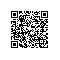# 1 前置知识点

Actor负责输出policy，也就是在某个状态下执行各种action的概率分布
Critic负责输出Vaue of state。
Actor和Critic的默契：Actor相信Critic给的状态的value就是真的； Critic也相信Actor选送过来的（s,a)中的a就是最优的action。通过不断的迭代，强化这个信任关系的正确性。
（这体现了我们的价值观 [因为信任，所以简单]，哈哈哈~）$$V_s=r_{s,a}+\gamma V_{s'}$$

$$Adv=r_{s,a}+\gamma V_{s'}-Vs$$

# 2 Proximal Policy Optimization（PPO）

## 2.2 PPO怎么解决这个问题的？

### 2.2.1 PPO-Clip

$$\theta_{k+1}=arg max_{\theta}E_{s,a \sim \pi_{\theta_k}}[L(s,a,\theta_k,\theta)]$$

$$L(s,a,\theta_k,\theta)=min \left( \frac{\pi_{\theta}(a|s)}{\pi_{\theta_k}(a|s)}A^{\pi_{\theta_k}}(s,a) , clip \left( \frac{\pi_{\theta}(a|s)}{\pi_{\theta_k}(a|s)}, 1-\epsilon, 1+\epsilon \right)A^{\pi_{\theta_k}}(s,a) \right)$$

$$L(s,a,\theta_k,\theta)=min \left( \frac{\pi_{\theta}(a|s)}{\pi_{\theta_k}(a|s)}A^{\pi_{\theta_k}}(s,a) , g \left( \epsilon, A^{\pi_{\theta_k}}(s,a) \ \right) \right)$$

g \left( \epsilon, A \right)=\left\{ \begin{aligned} &(1+\epsilon)A & A\ge 0 \\ &(1-\epsilon)A & A< 0 \end{aligned} \right.  当Advantage>=0的时候， $$L(s,a,\theta_k,\theta)=min \left( \frac{\pi_{\theta}(a|s)}{\pi_{\theta_k}(a|s)}, （1+\epsilon） \right)A^{\pi_{\theta_k}}(s,a)$$ 这就清楚的说明，这时候应该增大$\pi_{\theta}(a|s)$，也就是认为这个action是好的，增加选择$a$的概率。但是通过$1+\epsilon$ 限制增大的幅度。 同理，当Advantage<0的时候 $$L(s,a,\theta_k,\theta)=min \left( \frac{\pi_{\theta}(a|s)}{\pi_{\theta_k}(a|s)}, （1-\epsilon） \right)A^{\pi_{\theta_k}}(s,a)$$ 缩小$\pi_{\theta}(a|s)$，但是幅度不能小于$1-\epsilon$ 另外，根据我的理解，$\pi_{\theta_k}(a|s)$应该截断梯度，也就是反向传到的时候用不着去更新Old Actor的参数。在OpenAI Spinningup的代码（[https://github.com/openai/spinningup/blob/master/spinup/algos/pytorch/ppo/ppo.py](https://github.com/openai/spinningup/blob/master/spinup/algos/pytorch/ppo/ppo.py)）确实是这样处理的，但是在Tianshou的代码里([https://github.com/thu-ml/tianshou/blob/master/tianshou/policy/ppo.py](https://github.com/thu-ml/tianshou/blob/master/tianshou/policy/ppo.py))没有做截断，结果也OK，想来对于$\pi_{\theta}(a|s)$来说，$\pi_{\theta_k}(a|s)$就是一个scalar factor, 这个factor是变量还是静态值，也许影响不那么大，而且本轮迭代结束后$\theta_k$也会被覆盖掉，反向传导更新了也白搭。 到这里，其实说的都是如何更新Actor。 怎么更新Critic的参数呢？ $$L_c（s,a,r_{s,a},s'）=|r_{s,a}+V^{\pi_{\theta_k}}_{s'}-V^{\pi_{\theta}}|$$ 唯一的不同是target value是用Old Critic计算的，这也是DRL领域的常规操作了. 小结一下，PPO-Clip就是通过Clip操作来防止步子迈太大的。作者实验证明Clip的效果比Penalty好。 ### 2.2.2 PPO-Penalty $$L^{KLPEN}(\theta)=\frac{\pi_{\theta}(a|s)}{\pi_{\theta_k}(a|s)}A^{\pi_{\theta_k}}(s,a) -\beta KLD\left( \pi_{\theta}(*|s), \pi_{\theta_k}(*|s) \right)$$ 理解上上面的，这个理解起来也就容易了，就是增加一个新旧Policy差异的惩罚项，差异通过KL divergence来衡量 (PS: 如理解有误支持，欢迎批评指正~)使用钉钉扫一扫加入圈子
+ 订阅